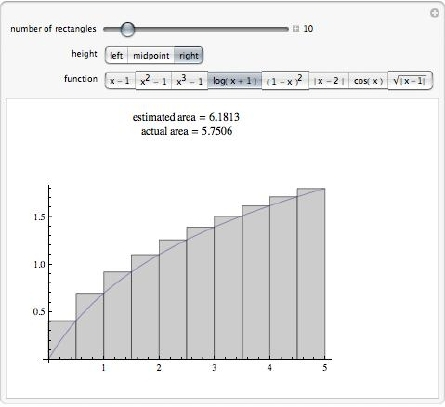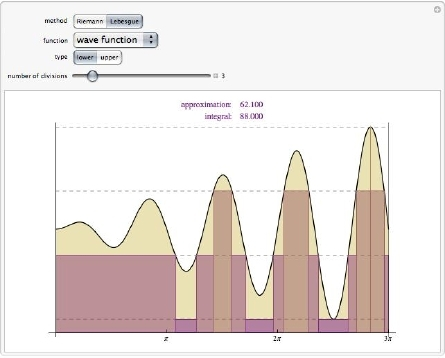Browse by Topic
Related Topics

# Visualizing Integrals

Calculus II is one of my favorite classes to teach, and the course I’ve probably taught more than any other. One reason for its special place in my heart is that it begins on the first day of class with a straightforward, easily stated, yet mathematically rich question: what is the area of a curved region? Triangles and rectangles—figures with straight sides—have simple area formulas whose derivation is clear. More complicated polygons can be carved up into pieces that are triangles and rectangles. But how does one go about finding the area of a blob?

After simplifying the blob to be a rectangle whose top side has been replaced with a curve, the stage is set for one of the classic constructions in calculus. The area of our simplified blob, reinterpreted as the area under the graph of a function is approximated using a series of rectangles. The approximation is obtained by partitioning the x axis, thus slicing the region into narrow strips, then approximating each strip with a rectangle and summing all the resulting approximations to produce a Riemann sum. Taking a limit of this process by using more and narrower rectangles produces the Riemann integral that forms the centerpiece of Calculus II. Several Demonstrations from the Wolfram Demonstrations Project, including “Riemann Sums” by Ed Pegg Jr, “Common Methods of Estimating the Area under a Curve” by Scott Liao and “Riemann Sums: A Simple Illustration” by Phil Ramsden show that this construction and images like the one below from “Riemann Sums” are part of the iconography of calculus.Early in graduate school, I learned of a different, more mysterious way of constructing integrals based on such seemingly esoteric notions as measurable sets and sigma fields. It took the better part of a yearlong course in analysis for me to appreciate the connection between this new, more general Lebesgue integral and my old friend the Riemann integral. The key, it turns out, was to think of the old area question.

The procedure outlined above approximates the curve that forms the top of the figure by a step function, i.e. a function that is constant on intervals. To construct a Lebesgue integral, begin instead by partitioning the y axis. The curve may now pass through one of the partition intervals multiple times, creating a “shadow” on the x axis that could be an interval, a combination of intervals or something more complicated. These shadows partition the x axis just as the original Riemann sum construction did but more intricately. You can define an approximating function by picking a single function value on each shadow—such a function is called a simple function. This construction leads to the Lebesgue integral and is beautifully illustrated by the Demonstration “Riemann versus Lebesgue” by Francisco J. Freniche. In the image below taken from this Demonstration, the dotted lines show the division of the y axis, and each color band on the x axis is one of the shadows.The Lebesgue integral turns out to be a generalization of the Riemann integral in the sense that if the Riemann approximations converge, so do the Lebesgue approximations and to the same value. There are, however, situations in which the Lebesgue approximations converge and the Riemann approximations do not. The Lebesgue integral is at the heart of measure theory and probability theory, and I had long thought of it as elegant but difficult to visualize. I still think of it as elegant.